# Niggli cell¶

## Usage of the code¶

The code is written in C and a python wrapper is prepared in python directory. The C code is used to compile with your code. Usual library interface is not prepared. The python code is used as a module. To use this, numpy is required.

In both C and python codes, there are two input arguments, lattice and eps. lattice is a double array with nine elements,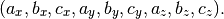In python, the input array will be fattened in the module. Therefore, e.g., the following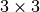shape of a numpy array or a python list is accepted: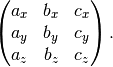The double variable of eps is used as the tolerance parameter. The value should be much smaller than lattice parameters, e.g., 1e-8. How it works is shown in the following section.

### Test¶

The test is found in python directory as a python code. A set of lattice parameters is found in lattices.dat and the references, which are the reduced lattice parameter made in the version 0.1.1, are stored in reduced_lattices.dat.

### Example¶

An example is found in python directory as a python code.

## Algorithm to determine Niggli cell¶

### Reference¶

1. A Unified Algorithm for Determining the Reduced (Niggli) Cell, I. Krivý and B. Gruber, Acta Cryst., A32, 297-298 (1976)
2. The Relationship between Reduced Cells in a General Bravais lattice, B. Gruber, Acta Cryst., A29, 433-440 (1973)
3. Numerically stable algorithms for the computation of reduced unit cells, R. W. Grosse-Kunstleve, N. K. Sauter and P. D. Adams, Acta Cryst., A60, 1-6 (2004)

### Algorithm¶

#### Update variables¶

The following variables used in this algorithm are initialized at the beginning of the algorithm and updated at the every end of A1-8 steps.

Define following variables as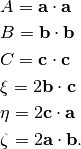They are elements of metric tensor where the off-diagonal elements are doubled. Therefore the metric tensor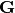is represented as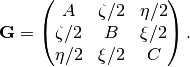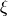,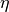,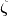are sorted by their ranges of angles as shown below.

Angle value
Acute 1
Obtuse -1
Right 0

These values are stored in variables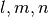as follows.

• Set initially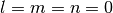.
• If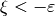,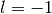.
• If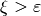,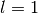.
• If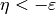,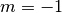.
• If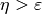,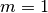.
• If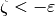,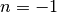.
• If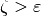,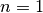.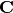found in each step is the transformation matrix that is applied to basis vectors: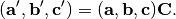#### A1¶

If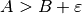or (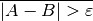and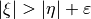),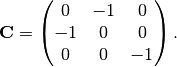#### A2¶

If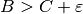or (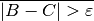and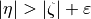),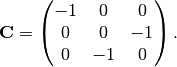Go to A1.

#### A3¶

If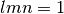:

•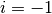ifelse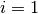•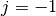ifelse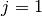•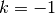ifelse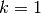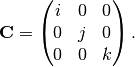#### A4¶

If,, and, do nothing in A4.

If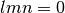or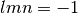:

Set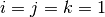.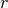is used as a reference to,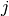, or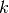, and is initially undefined.

•if•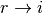if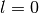•if•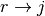if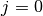•if•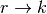if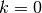If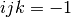:

•,, orrefered byis set to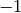.#### A5¶

If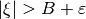or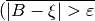and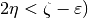or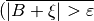and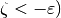: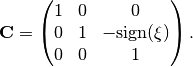Go to A1.

#### A6¶

If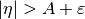or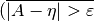and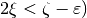or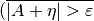and: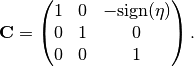Go to A1.

#### A7¶

If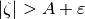or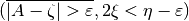or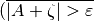and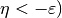: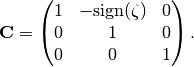Go to A1.

#### A8¶

If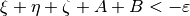or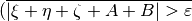and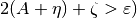: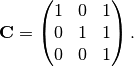Go to A1.